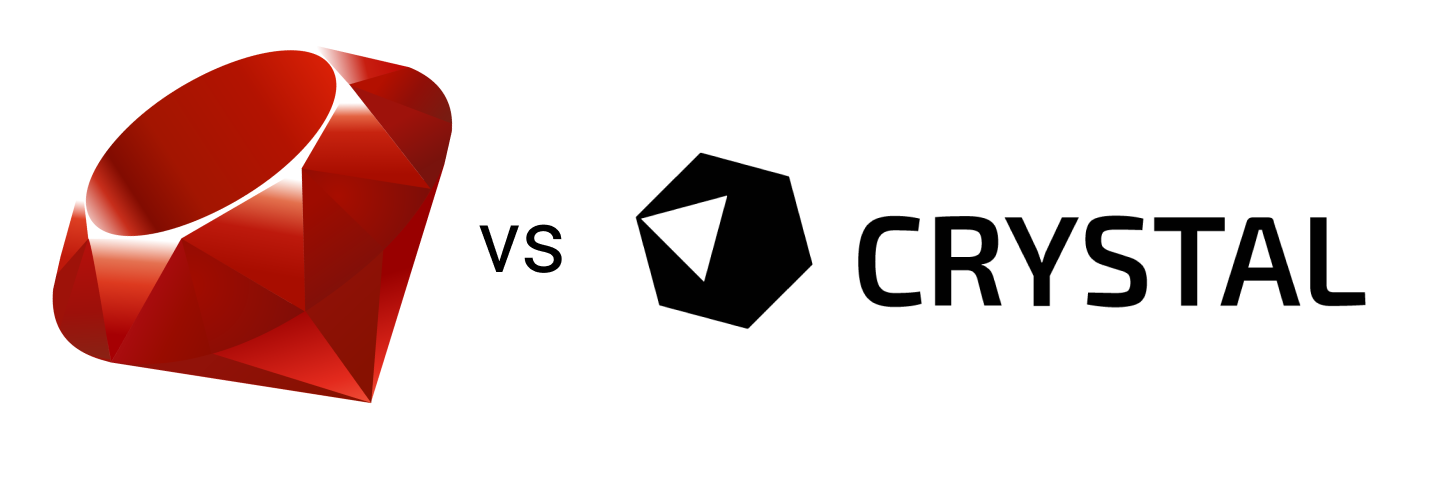# Ruby vs Crystal Performance

Pavel Timofeev · May 22, 2020You can join the discussion on HackerNews here.

I’ve been hearing about the Crystal programming language here and there over the last couple of years but never had a chance to give it a look until yesterday.

What is Crystal? It’s a statically typed, compiled, object-oriented language with syntax heavily inspired by Ruby’s.

The promise on its site is that the language is as fast as C, sleek as Ruby. This statement sounds exciting and makes you want to check how fast Crystal is comparing to Ruby. Of course, it won’t be a fair comparison since one is a compiled language and another is an interpreted one (the Ruby MRI implementation used below).

The installation process on MacOS is trivial with Homebrew:

``````brew install crystal
``````

To compile and run a Crystal program:

``````crystal program.cr
``````

Or you can compile it first and run afterwords with:

``````crystal build program.cr
./program
``````

An implementation of a basic HTTP server taken from the Crystal’s web site works as expected and the code indeed looks like Ruby’s:

``````require "http/server"

server = HTTP::Server.new do |context|
context.response.content_type = "text/plain"
context.response.print "Hello world, got #{context.request.path}!"
end

puts "Listening on http://127.0.0.1:8080"
server.listen(8080)
``````

## Performance Comparison

Let’s write some code in Ruby and Crystal to generate a Fibonacci sequence for a given number. We can then see how much time it takes to find the 46th number (which is 1,836,311,903) in the sequence. You will see in a bit why I picked this number.

Ruby:

``````def fibonacci(n)
return n if n < 2
fibonacci(n-1) + fibonacci(n-2)
end

puts fibonacci(46)
``````

Crystal’s code is identical:

``````def fibonacci(n)
return n if n < 2
fibonacci(n-1) + fibonacci(n-2)
end

puts fibonacci(46)
``````

Results on my machine (MacBook Pro 2.2 GHz Intel Core i7):

 Language Run time Memory usage Ruby 2.6.5p114 (2019-10-01 revision 67812) 1:54.46 minutes 16.1M Crystal 0.34.0 (2020-04-07) 12.617 seconds 1.87M

The Crystal version is 11 times faster.

If we try to find the next, 47th number in the Fibonacci sequence, Crystal will actually give us an error:

``````Unhandled exception: Arithmetic overflow (OverflowError)
from fibonacci
from fib.cr:6:6 in '__crystal_main'
from /usr/local/Cellar/crystal/0.34.0/src/crystal/main.cr:105:5 in 'main'
``````

What happens here?

If you recall Crystal is a statically typed language but you can omit explicit type restriction and the compiler will try to infer the type of variable. In our code Crystal uses the Int32 type for the n variable which has the maximum value of 2,147,483,647 but the 47th number is higher. In this case we need to specify the type of n. We can use Unsigned Int 64.

``````def fibonacci(n : UInt64)
return n if n < 2
fibonacci(n-1) + fibonacci(n-2)
end

puts fibonacci(47)
``````

Now the code works as expected.

## Code Optimization

We can optimize our code and introduce memoization to return cached results of the previously calculated numbers.

Ruby:

``````def fibonacci(n, cache = [0, 1])
return cache[n] if cache[n]
cache[n] = fibonacci(n-1, cache) + fibonacci(n-2, cache)
end

puts fibonacci(100)
``````

The version for Crystal looks slightly different:

``````def fibonacci(n, cache = [0, 1] of UInt128)
return cache[n] if cache[n]?
(cache << fibonacci(n-1, cache) + fibonacci(n-2, cache))[-1]
end

puts fibonacci(100)
``````

When we try to find the 100th number in the Fibonacci sequence which is pretty big: 354,224,848,179,261,915,075 we end up with the following results:

 Ruby The run time is 0.272 seconds. Crystal The run time is 0.009 seconds.

Which makes the point that what has to be optimized first is the algorithm and not picking up a faster language or machine.

## Conclusion

The language looks interesting and promising. Familiar syntax, great performance, fantastic documentation.